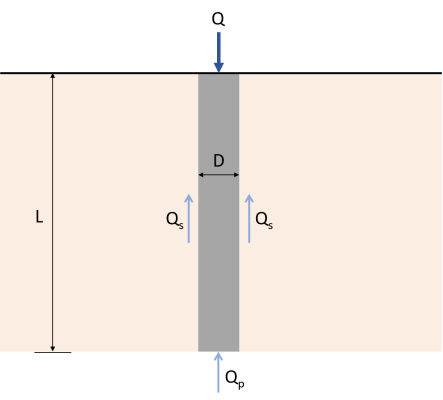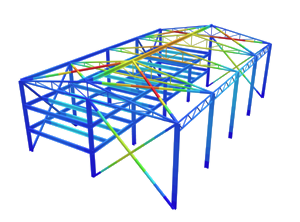SkyCiv Documentation

Your guide to SkyCiv software - tutorials, how-to guides and technical articles

1. Home
2. SkyCiv Foundation
3. Piles

## How to calculate the ultimate load-carrying capacity of a single pile

Evaluating the ultimate load-carrying capacity of a single pile is one of the most important aspects of pile design, and can sometimes be complicated. This article will walk through the governing equations for single pile design as well as an example.

To easily understand the load transfer mechanism of a single pile, imagine a concrete pile of length L with diameter D, as shown in Figure 1.Figure 1: Load transfer Mechanism for piles

The load Q applied on the pile shall be transferred directly to the soil at the bottom of the pile. Part of this load will be resisted by the sides of the pile using something called “skin friction” developed along the shaft (Qs), and the rest will be resisted by the soil that the pile is bearing on (Qp).  Therefore, the ultimate load-carrying capacity (Qu) of a pile shall be given by the equation (1). There are multiple methods available to estimate the values of Qp and Qs.

$${Q}_{u} = {Q}_{p} + {Q}_{s}$$    (1)

Qs = Skin-frictional resistance

Want to try SkyCiv’s Foundation Design software? Our free tool allows users to perform load-carrying calculations without any download or installation!

## End-bearing Capacity, Qp

Ultimate end-bearing capacity is theoretically the maximum load per unit area that can be supported by the soil in bearing, without failure. The following equation of Karl Von Terzaghi, the father of soil mechanics, is one of the first and most commonly used theory when evaluating the ultimate bearing capacity of foundations. Terzaghi’s equation for ultimate bearing capacity can be expressed as:

$${q}_{u} = (c × {N}_{c}) + (q × {N}_{q}) + (\frac{1}{2} × γ × B × {N}_{γ})$$    (2)

qu = Ultimate end-bearing capacity

c = Cohesion of soil

q = Effective soil pressure

γ = Soil unit weight

B = Cross-sectional depth or diameter

Nc, Nq, Nγ = Bearing factors

Since qu is in terms of load per unit area or pressure, multiplying it by the cross-sectional area of the pile will result in the end-bearing load capacity (Qp) of the pile. The resulting value of the last term of Equation 2 is negligible due to a relatively small pile width, hence,  it may be dropped from the equation. Thus, the ultimate end-bearing load capacity of the pile can be expressed as shown in equation (3). This modified version of Terzaghi’s equation is used in the SkyCiv Foundation module when designing piles.

$${Q}_{p} = {A}_{p} × [(c × {N}_{c}) + (q × {N}_{q}) ]$$    (3)

Ap = Cross-sectional area of pile

Bearing factors Nand Nare non-dimensional, empirically derived, and are functions of the soil friction angle (Φ). Researchers have already completed the calculations required to find bearing factors. Table 1 summarizes the values of Naccording to Naval Facilities Engineering Command (NAVFAC DM 7.2, 1984). The value of Nc is approximately equal to 9 for piles under clayey soils.

Bearing Factor (Nq)
Friction Angle (Ø) 26 28 30 31 32 33 34 35 36 37 38 39 40
Driven Piles 10 15 21 24 29 35 42 50 62 77 86 120 145
Bored Piles 5 8 10 12 14 17 21 25 30 38 43 60 72

Table 1: Nvalues from NAVFAC DM 7.2

## Skin-frictional Resistance Capacity, Qs

Skin-frictional resistance of piles is developed along the length of the pile. Generally, the frictional resistance of a pile is expressed as:

$${Q}_{s} = ∑ (p × ΔL × f)$$    (4)

p = Perimeter of the pile

ΔL = Incremental pile length over which p and f are taken

f = Unit frictional resistance at any depth

Estimating the value of the unit frictional resistance (f) requires several important factors to consider, such as the nature of pile installation and soil classification. Equations (5) and (6) shows the computational method to find the unit frictional resistance of piles in sandy and clayey soils, respectively. Tables 2 and 3 present the recommended effective earth pressure coefficient (K) and the soil-pile frictional angle (δ’), according to NAVFAC DM7.2.

For sandy soils:

$$f = K × σ’× tan(δ’)$$    (5)

K = Effective earth pressure coefficient

σ’ = Effective vertical stress at the depth under consideration

δ’ = Soil-pile frictional angle

For clayey soils:

$$f = α × c$$    (6)

Soil-Pile Frictional Angle (δ’)
Pile Type δ’
Steel Pile 20º
Timber Pile 3/4 × Φ
Concrete Pile 3/4 × Φ

Table 2: Soil-Pile Frictional Angle Values (NAVFAC DM7.2, 1984)

Lateral Earth Pressure Coefficient (K)
Pile Type Compression Pile Tension Pile
Driven H-piles 0.5-1.0 0.3-0.5
Driven displacement piles (round, rectangular) 1.0-1.5 0.6-1.0
Driven displacement piles (tapered) 1.5-2.0 1.0-1.3
Driven jetted piles 0.4-0.9 0.3-0.6
Bored piles (<24″ Diameter) 0.7 0.4

Table 3: Lateral Earth Pressure Coefficient (K) Values (NAVFAC DM7.2, 1984)

c/pa α
≤ 0.1 1.00
0.2 0.92
0.3 0.82
0.4 0.74
0.6 0.62
0.8 0.54
1.0 0.48
1.2 0.42
1.4 0.40
1.6 0.38
1.8 0.36
2.0 0.35
2.4 0.34
2.8 0.34

Note: p= atmospheric pressure ≈ 100 kN/m2

Table 4: Adhesion Factor Values (Terzaghi, Peck, and Mesri, 1996)

## Example: Calculating the capacity of piles in sand

A 12-meter long concrete pile with a diameter of 500 mm is driven into multiple sand layers with no groundwater present. Find the ultimate load-carrying capacity (Qu)  of the pile.

Details
Section
Diameter 500 mm
Length 12 m
Layer 1-Soil Properties
Thickness 5 m
Unit Weight 17.3 kN/m3
Friction Angle 30 Degrees
Cohesion 0 kPa
Groundwater Table Not present
Layer 2-Soil Properties
Thickness 7 m
Unit Weight 16.9 kN/m3
Friction Angle 32 Degrees
Cohesion 0 kPa
Groundwater Table Not present

Step 1: Compute the end-bearing load capacity (Qp).

At the tip of the pile:

Ap = (π/4) × D2 = (π/4) × 0.52

Ap = 0.196 m2

c = 0 kPa

θ = 32º

N= 29 (From Table 1)

Effective soil pressure (q):

q =  (γ × t1) +  (γ × t2) =  (5 m × 17.3 kN/m3) +  (7 m × 16.9 kN/m3)

q = 204.8 kPa

Then use equation (3) for the end-bearing load capacity:

Qp = Ap × [(c × Nc) + (q × Nq)]

Qp = 0.196 m× ( 204.8 KPa × 29)

Q= 1,164.083 kN

Step 2:  Compute the skin-frictional resistance (Qs).

Using equations (4) and (5), calculate the skin-frictional per soil layer.

Qs = ∑ (p × ΔL ×  f)

p = π × D = π × 0.5 m

p = 1.571 m

Layer 1:

ΔL = 5 m

f1 = K × σ’1× tan(δ’)

K = 1.25 (Table 3)

δ’ = 3/4 × 30º

δ’ = 22.50º

σ’1 = γ × (0.5 × t1)  = 17.3 kN/m3 × (0.5 × 5 m)

σ’1 = 43.25 kN/m2

f1 = 1.25 × 43.25 kN/m2 × tan(22.50º)

f1 = 22.393 kN/m2

Qs1 = p × ΔL ×  f1 = 1.571 m ×5 m × 22.393 kN/m2

Qs1 = 175.897 kN

Layer 2:

ΔL = 7 m

f2 = K × σ’2× tan(δ’)

K = 1.25 (Table 3)

δ’ = 3/4× 32º

δ’ = 24º

σ’2 = (γ × t1) + [γ × (0.5 × t2)]  = (17.3 kN/m3 × 5 m) + [16.9 kN/m3 ×(0.5 × 7 m)]

σ’2 = 145.65 kN/m2

f2 = 1.25 × 145.65 kN/m2 × tan(24º)

f2 = 81.059 kN/m2

Qs2 = p × ΔL ×  f2 = 1.571 m ×7 m × 81.059 kN/m2

Qs2 = 891.406 kN

Total skin-frictional resistance:

Q= Qs1+ Qs2 = 175.897 kN + 891.406 kN

Qs = 1,067.303 kN

Step 3:  Compute for the ultimate load-carrying capacity (Qu).

Q= Qp+ Q= 1,164.083 kN + 1,067.303 kN

Qu = 2,231.386 kN

## Example 2: Calculating the capacity of piles in clay

Consider a 406 mm diameter concrete pile with a length of 30m embedded in layered, saturated clay. Find the ultimate load-carrying capacity (Qu)  of the pile.

Details
Section
Diameter 406 mm
Length 30 m
Layer 1-Soil Properties
Thickness 10 m
Unit Weight 8 kN/m3
Friction Angle
Cohesion 30 kPa
Groundwater Table 5 m
Layer 2-Soil Properties
Thickness 10 m
Unit Weight 19.6 kN/m3
Friction Angle
Cohesion 0 kPa
Groundwater Table Fully submerged

Step 1: Compute the end-bearing load capacity (Qp).

At the tip of the pile:

Ap = (π/4) × D2= (π/4) × 0.4062

Ap = 0.129 m2

c = 100 kPa

N= 9 (Typical value for clay)

Q= (c × Nc) × A= (100 kPa × 9) × 0.129 m2

Q= 116.1 kN

Step 2: Compute the skin-frictional resistance (Qs).

Using equations (4) and (6), calculate the skin-frictional per soil layer.

Q = ∑ (p × ΔL ×  f)

p = π × D = π × 0.406 m

p = 1.275 m

Layer 1:

ΔL = 10 m

α= 0.82 (Table 4)

c= 30 kPa

f1=  α1 × c1  = 0.82 × 30 kPa

f= 24.6 kN/m2

Qs1  = p × ΔL ×  f1 = 1.275 m × 10 m × 24.6 kN/m2

Qs1 = 313.65 kN/m2

Layer 2:

ΔL = 20 m

α2= 0.48 (Table 4)

c= 100 kPa

f =  α2 × c = 0.48 × 100 kPa

f= 48 kN/m2

Qs2  = p × ΔL ×  f = 1.275 m × 20 m × 48 kN/m2

Qs2 = 1,224 kN/m2

Total skin-frictional resistance:

Q= Qs1+ Qs2 = 313.65 kN + 1224 kN

Qs = 1,537.65 kN

Step 3:  Compute for the ultimate load-carrying capacity (Qu).

Q= Qp+ Q= 116.1 kN + 1537.65 kN

Qu = 1,653.75 kN

Want to try SkyCiv’s Foundation Design software? Our free tool allows users to perform load-carrying calculations without any download or installation!

### References:

• Das, B.M. (2007). Principles of Foundation Engineering (7th Edition). Global Engineering
• Rajapakse, R. (2016). Pile Design and Construction Rule of Thumb (2nd Edition). Elsevier Inc.
• Tomlinson, M.J. (2004). Pile Design and Construction Practice (4th Edition). E & FN Spon.